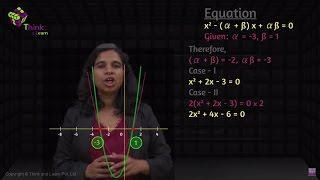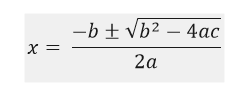A quadratic equation is a polynomial whose highest exponent is the square of a variable. Standard form of this equation looks like ax2 + bx + c = 0, where a ≠ 0 and a, b, c are numbers. The solution for this equation is based on the roots of the equation. There are many exercise and word problems which can be resolved with the help of this concept.

Students can also download the PDF of quadratic equation to understand the concept in a better way. This is one of the most important topics in maths and the key points that are covered here are:

• Definition
• Standard form
• Video Lesson
• Solutions
• Formula and Discriminant
• Nature of Roots of the 2nd order Equation
• Applications

Definition

A quadratic equation is defined as an equation which has a degree of 2, also called an “equation of degree 2”. The word “Quadratic” comes from “quad” means four and “Quadratic” means ‘to make a square’.

Standard Form

The standard form of a polynomial equation having a degree two is ax2 + bx + c = 0, a ≠ 0 Where “x” is a variable and a, b, c are real numbers.

Note: If a = 0 then the equation will become a linear equation.

Video LessonFormula

Every equation is solved to find the values of the variable. Similarly, we use here, Sridhara’s Method, one of the most common methods for finding the roots.

Let ax2 + bx + c = 0, is the given equation, such that:Here, “±” represent both the values (one positive and one negative), hence there are two possible roots for the equation.

Depending upon the value of discriminant, the equation with degree 2 can have 3 different types of roots, namely:

S.no Value of Discriminant (D) Nature of Roots
1 b2 – 4ac > 0 Two distinct Real roots
2 b2 – 4ac >= 0 Two equal roots
3 b2 – 4ac < 0 Two distinct Complex Roots

Solution

As a quadratic equation has degree of 2, hence the number of solution that will satisfy the given equation will also be equal to 2. There can be more than one method to solve this equation:

• Factorisation
• Sridhara’s Formula
• Harriot’s Method

Examples:

 Example 1: Find the roots of the equation 2x2 + 7x + 3 = 0 Solution: Given: 2x2 + 7x + 3 = 0 Here, a = 2, b = 7 and c = 3 Finding the value of D, D = b2 − 4ac = 72 – 4 × 2 × 3 = 49 – 24 ⇒D = 25 As D > 0, the equation has two distinct real roots. $x = \frac{-b \pm \sqrt{D}}{2a}$ ⇒x = -7  ± √25/2 × 2 ⇒ x = -7 ± 5/4 ⇒x = -7 – 5/4 or -7 + 5/4 ⇒x = -3 or -1/2 Example 2: Find the roots of the equation: x2 + 2x – 3 = 0 Solution: Given equation is x2 + 2x – 3 = 0 Let’s use the factorization method to find its roots x2 – x + 3x – 3 = 0 x(x – 1) + 3(x – 1) = 0 (x – 1)(x + 3) = 0 Therefore, x = 1 or x = -3 are the roots of the given equation.

Applications

Quadratic equations are used to represent many mathematical and physical problems. A parabola is one of the real examples of it.

These equations help to find the position of an object travels in the air and again hit the surface back, loss or profit for items sold, speed and distance covered by a boat in the water against upstream or downstream and many more.

Word Problems

Problem 1: Vicky agreed to paint a wall for Rs. 150. It took him two hours longer than he expected, and therefore he earned Rs. 2.50 per hour less than anticipated. How long did he expect the painting would take?

Solution:

Let m be the number of hours he expected. Then m+2 the total number of hours actually took to complete the painting. As he earned Rs. 2.50 per hour less. The rate of pay is divided by the number of hours.

Using above details, we have equation

150/x – 5/2 = 150/(x+2)

(300 – 5x)/2x = 150/(x+2)

300(x+2) – 5x(x+2) = 300x

300 x + 600 – 5x2 – 10x = 300x

-5x2 – 10x – 600 = 0

x2 + 2x – 120 = 0

(x + 12)(x – 10) = 0

or x = -12 or x = 10

Ignore the negative value as it is not valid. Therefore he anticipated that the painting would take 10 hours.

Problem 2: Krishan bought a land whose length is 10 times the width. If the land is expanded in the 1000 square meter area. What would be the length and width of the land?

Solution: Let x be the width of the land. Then 10x be its length. Also, we are given with area of the land which is 1000 m2.

Therefore, 10x2 = 1000

x2  = 100

x = 10 or x = -10

Ignore negative value as length cannot be negative.

Therefore, the width is 10 m and length is 10(10) = 100 m.

Download BYJU’S – The Learning App and get personalized videos explaining the mathematical concepts by our experts.